##### Circuit Analysis For Dummies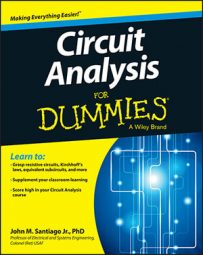Laplace transform methods can be employed to study circuits in the s-domain. Laplace techniques convert circuits with voltage and current signals that change with time to the s-domain so you can analyze the circuit's action using only algebraic techniques.

Connection constraints are those physical laws that cause element voltages and currents to behave in certain ways when the devices are interconnected to form a circuit. You also have constraints on the individual devices themselves, where each device has a mathematical relationship between the voltage across the device and the current through the device. Here you learn what connection constraints, device constraints, impedances, and admittances look like in the s-domain.

## Connection constraints in the s-domain

Transforming the connection constraints to the s-domain is a piece of cake. Kirchhoff’s current law (KCL) says the sum of the incoming and outgoing currents is equal to 0. Here’s a typical KCL equation described in the time-domain:

i1(t) + i2(t) – i3(t) = 0

Because of the linearity property of the Laplace transform, the KCL equation in the s-domain becomes the following:

I1(s) + I2(s) – I3(s) = 0

You transform Kirchhoff’s voltage law (KVL) in the same way. KVL says the sum of the voltage rises and drops is equal to 0. Here’s a classic KVL equation described in the time-domain:

v1(t) + v(t) + v3(t) = 0

Because of linearity, the KVL equation in the s-domain produces

V1(s) + V2(s) + V3(s) = 0

The basic form of KVL remains the same. Piece of cake!

## Device constraints in the s-domain

You can easily transform the i-v constraints of devices such as independent and dependent sources, op amps, resistors, capacitors, and inductors to algebraic equations in the s-domain. After converting the device constraints, all you need is algebra to translate current and voltage relationships to the s-domain.

Transforming independent sources is a no-brainer because the s-domain has the same form as the time-domain: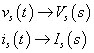Converting dependent sources is easy, too. Here are the equations for voltage-controlled voltage sources (VCVS), voltage-controlled current sources (VCCS), current-controlled voltage sources (CCVS), and current-controlled current sources (CCCS):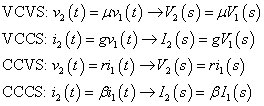The constants μ, g, r, and β relate the dependent output sources V2(s) and I2(s) controlled by input variables V1(s) and I1(s).

For resistors, capacitors, and inductors, you convert their i-v relationships to the s-domain using Laplace transform properties, such as the integration and derivative properties: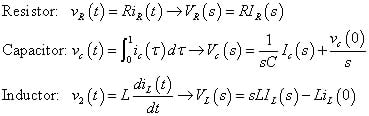The preceding three equations on the right are s-domain models that use voltage sources for the initial capacitor voltage vC(0) and initial inductor current iL(0).

You can rewrite these equations in the s-domain to model the initial conditions, vC(0) and iL(0), as current sources: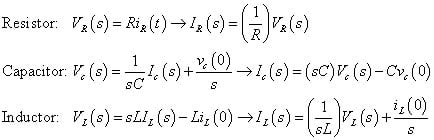You see there are no integrals or derivatives in the s-domain.

The middle column here shows the constraints of the passive devices in the time-domain being converted to the s-domain. The left column shows initial conditions modeled as voltage sources in the s-domain, and the right column shows initial conditions modeled as current sources in the s-domain.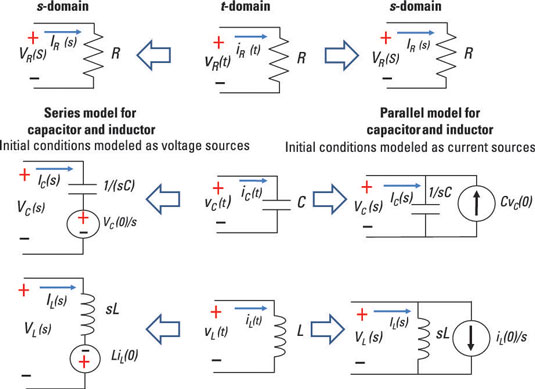Taking the initial conditions into account in the s-domain analysis for capacitors and inductors is a big deal because it expedites the analysis. When you transform differential equations into the s-domain, you deal with input sources and initial conditions simultaneously.

The constraints of ideal operational amplifiers are unchanged in form in the s-domain: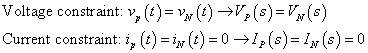Impedance Z relates the voltage and current described in the s-domain when initial conditions are set to 0. The following algebraic form of the i-v relationship describes impedance in the s-domain:

V(s) = Z(s)I(s)

Admittance Y is the reciprocal of the impedance; it’s useful when you’re analyzing parallel circuits: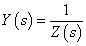In the s-domain for zero initial conditions, the element constraints, impedances Z(s), and admittances Y(s) for the passive devices are as follows: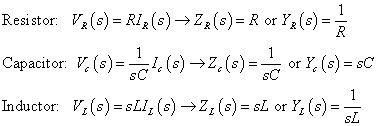Now you’re ready to start analyzing circuits in the s-domain — without having to rely on calculus.